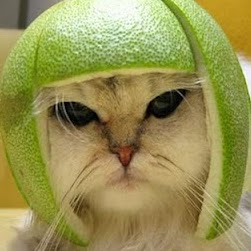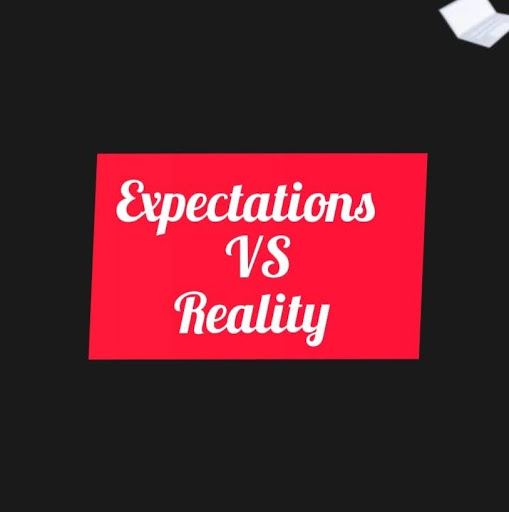# Three partners A , B , C start a business . B's Capital is four times C's capital and twice A's capital is equal to thrice B's capital . If the total profit is Rs 16500 at the end of a year ,Find out B's share in it.

A. Rs. 4000

B. Rs. 5000

C. Rs. 6000

D. Rs. 7000

### Solution(By Examveda Team)

Suppose C's capital = x then
B's capital = 4x (Since B's Capital is four times C's capital)
A's capital = 6x ( Since twice A's capital is equal to thrice B's capital)
A : B : C =6x : 4x : x
= 6 : 4 : 1
B's share = $$16500 \times \frac{4}{{11}}$$   = 1500 × 4 = 6000.

1.2A=3B
A:B=3:2
B=4C
B:C=4:1
A:B:C=6:4:1
B's share is 16500*(4/11)=6000

2.I think the word "twice" was wrongly inserted in the second line of question.

The answer provided by exam Veda will be correct only if we eliminate that "twice" word from the second line.

3.Here Twice A capital is equal to 3B capital
As we know B capital=4x
So as per question statement
2A=3B
2A=3(4x)
So A=12x/2
A=6x

4.Suppose C's capital = x then
B's capital = 4x (Since B's Capital is four times C's capital)
A's capital = 12x ( Since twice A's capital is equal to thrice B's capital)
A:B:C = 12x : 4x : x
= 12 : 4 : 1
B's share = 16500 * (4/17) = 1500*4 = 3882.35

It's correct right?

5.Hey..! B's capital = 4X
So, A's capital = 4X(3) = 12X ( Since twice A's capital is equal to thrice B's capital)

6.what is role of number 11, is this month or any other thing??

7.B=4x
A=4x*3=12x?????
isnt it??

Related Questions on Partnership